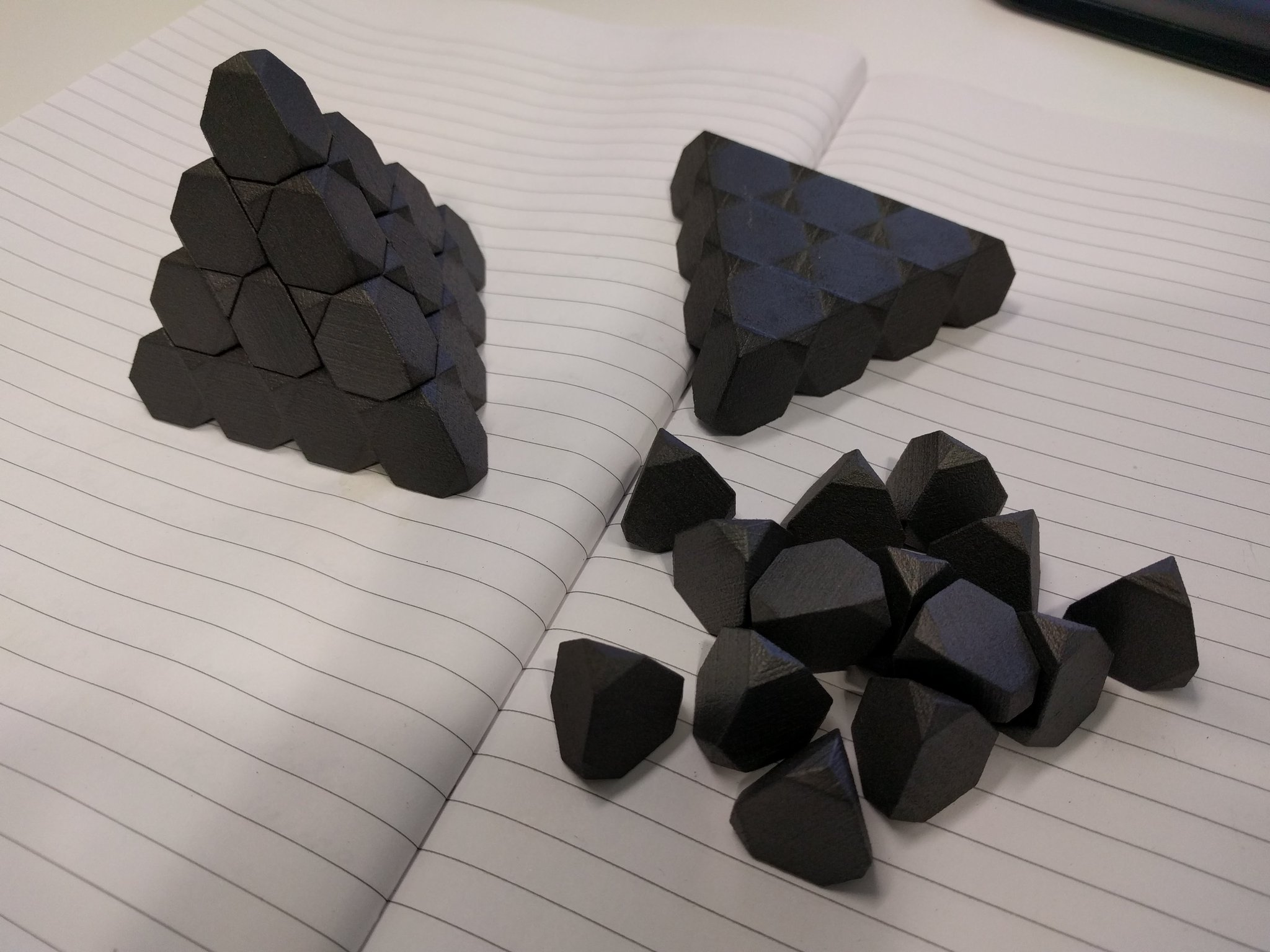# Diamonds

Tim Hutton recently presented me with a marvellous 3D-printed-graphite set of triakis truncated tetrahedra. These have a natural interpretation as the Voronoi cells of a diamond: the shapes that would form if you gradually enlarged the atoms until they tessellate the ambient space.He also produced a video describing the relationship between the Voronoi cells of the diamond and related lattices.

### Hyperdiamonds

Mathematically, the set of centres of atoms in a diamond form a structure called$D_3^+$. It has a natural n-dimensional generalisation, which can be viewed as follows:

• Take the integer lattice$\mathbb{Z}^n$;
• Partition it into two subsets, called E and O, consisting of the points whose coordinate-sums are even and odd, respectively;
• Translate the set O such that it contains the point (½, ½, ½, …, ½), and leave E where it is.

This construction makes it adamantly* clear that the ‘hyperdiamond’ has the same density as the integer lattice, and therefore the Voronoi cells have unit volume. A lattice with this property (such as the hyperdiamonds of even dimension) is described as unimodular.

*bizarrely, this word has the same etymology as ‘diamond’, namely the Greek word adamas meaning ‘indestructible’. I often enjoy claiming that my own given name is also derived from this same etymology.

In the special case where n = 8, this is the remarkable E8 lattice, which Maryna Viazovska proved is the densest way to pack spheres in eight-dimensional space. One could imagine that if beings existed in eight-dimensional space, they would have very heavy diamond engagement rings! It is an even unimodular lattice, meaning that the inner product of any two vectors is an even integer, and is the unique such lattice in eight dimensions. They can only exist in dimensions divisible by 8, and are enumerated in A054909.

If n = 16, the hyperdiamond gives one of two even unimodular 16-dimensional lattices, the other being the Cartesian product of E8 with itself. These two lattices have the same theta series (sequence giving the number of points at each distance from the origin), but are nonetheless distinct. If you take the quotients of 16-dimensional space by each of these two lattices, you get two very similar (but non-isometric) tori which Milnor gave as a solution to the question of whether two differently-shaped drums could produce the same sound when reverberated.

John Baez elaborates on the sequence of ‘hyperdiamonds’, together with mentioning an alternative three-dimensional structure called the triamond, here.

### Niemeier lattices

There are 24 even unimodular lattices in 24 dimensions, the so-called Niemeier lattices, of which the most famous and stunning is the Leech lattice. This was also proved by Viazovska and collaborators to be the densest sphere packing in its dimension. Unlike the hyperdiamonds, however, the Leech lattice is remarkably complicated to construct.

Whereas the shortest nonzero vectors in the Leech lattice have squared norm of 4, the other 23 Niemeier lattices have roots (elements of squared norm 2). The Dynkin diagrams of these root systems identify the lattices uniquely, and conversely all Dynkin diagrams with certain properties give rise to Niemeier lattices.

The 23 other Niemeier lattices correspond exactly to the equivalence classes of deep holes in the Leech lattice, points at a maximum distance from any of the lattice points.

This entry was posted in Uncategorized. Bookmark the permalink.

### 5 Responses to Diamonds

1.Jeffrey Ventrella says:

Hello Adam, interesting article as usual!

I especially like seeing the relationship of the cube, the triakis truncated tetrahedron, and the rhombic dodecahedron as the three space-filling volumes found in the structure of diamond. I suspect the cube is the only (recursively) tile-able volume of the three. This is based on my findings after exploring the possibility of recursive space-filling with rhombic dodecahedra:

https://spacefillingcurves.wordpress.com/2016/09/27/is-there-a-3d-gosper-curve/

I was wondering if you may have a mathematically rigorous way of explaining why it is not possible to (recursively) fill space with 13^n rhombic dodecahedra (constructing a self-similar 3D “fractile” – as shown in my blog post). The 2D analogy would be recursively filling the plane with 7^n hexagons, as is often illustrated in relation to the Gosper curve.

I am wondering if this problem is related to the nature of 3 dimensional space. You have written about lattices in higher dimensions, and you advised me to consider space-filling curves as complex integers in 2D lattices, and so I wonder if the quaternion space would be rich with more possibilities? 🙂

Thanks!
-Jeffrey

•Dan Asimov says:

“… 13^n rhombic dodecahedra …”

Is it possible this was meant to be 15^n (because a rhombic dodecahedron has 14 faces)?

•Jeffrey Ventrella says:

The rhombic dodecahedron has 12 faces, hence it’s name. If you start with one in the center and surround it with 12 (one for each face) that makes 12. It is analogous to surrounding a hexagon with six hexagons to make 7.

2.Liang Zhao says:

Hi Adam: if I want to construct a truncated or bi-truncated or even omni-truncated regular polytope, say the truncated cube, and I have its symmetric group and vertex/edge/face stabilizing subgroup at hand (knowing the multiplication of the coset table by generators of the Coxeter group), how can I construct all vertices and edges and faces?

•Volker says:

This might not be the best place to ask math questions. I strongly recommend MathOverflow, where not only Adam is active, but many other mathematicians as well:

https://mathoverflow.net/